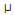# Electronics - Bipolar Junction Transistors (BJT)

### Exercise :: Bipolar Junction Transistors (BJT) - General Questions

11.

The C-B configuration is used to provide which type of gain?

 A. voltage B. current C. resistance D. power

Explanation:

No answer description available for this question. Let us discuss.

12.

The Q point on a load line may be used to determine:

 A. VC B. VCC C. VB D. IC

Explanation:

No answer description available for this question. Let us discuss.

13.

A transistor may be used as a switching device or as a:

 A. fixed resistor B. tuning device C. rectifier D. variable resistor

Explanation:

No answer description available for this question. Let us discuss.

14.

If an input signal ranges from 20–40A (microamps), with an output signal ranging from .5–1.5 mA (milliamps), what is the ac beta?

 A. 0.05 B. 20 C. 50 D. 500

Explanation:

No answer description available for this question. Let us discuss.

15.

Which is beta's current ratio?

 A. IC/IB B. IC/IE C. IB/IE D. IE/IB